# Frank solutions for Class 9 Physics ICSE chapter 6 - Light [Latest edition]

#### Chapters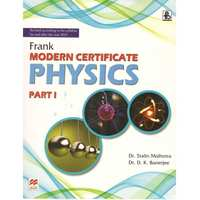## Chapter 6: Light

Reflection of LightExerciseSpherical Mirrors
Reflection of Light, Exercise [Pages 245 - 247]

### Frank solutions for Class 9 Physics ICSE Chapter 6 Light Reflection of Light, Exercise [Pages 245 - 247]

Reflection of Light | Q 1 | Page 245
What do you mean by light?
Reflection of Light | Q 2 | Page 245
Is light a form of energy?
Reflection of Light | Q 3 | Page 245
What is the velocity of light in a vacuum?
Reflection of Light | Q 4 | Page 245
Name two common sources of light.
Reflection of Light | Q 5 | Page 245
Write down four characteristics of light.
Reflection of Light | Q 6 | Page 245
Name two luminous bodies.
Reflection of Light | Q 7 | Page 245
Name two non-luminous bodies.
Reflection of Light | Q 8 | Page 245
Distinguish between convergent and divergent beams of light.
Reflection of Light | Q 9 | Page 245
What is meant by a ray of light?
Reflection of Light | Q 10 | Page 245
Give three distinctions between sound and light waves.
Reflection of Light | Q 11.1 | Page 246

Identify the following kind of beam of light.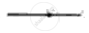Reflection of Light | Q 11.2 | Page 246

Identify the following kind of beam of light.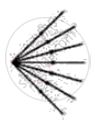Reflection of Light | Q 11.3 | Page 246

Identify the following kind of beam of light.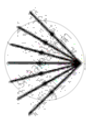Reflection of Light | Q 11.4 | Page 246

Identify the following kind of beam of light.Reflection of Light | Q 12 | Page 246
Name the substance through which light is made to pass.
Reflection of Light | Q 13 | Page 246
What is meant by rectilinear propagation of light?
Reflection of Light | Q 14 | Page 246
Is glass an opaque medium?
Reflection of Light | Q 15 | Page 246
Are metals transparent by nature?
Reflection of Light | Q 16 | Page 246
What is the difference between a ray of light and a beam of light?
Reflection of Light | Q 17 | Page 246

Out of the following, choose the substance which is translucent medium:

• Wood

• water

• waxed paper

• stone

• black paper

Reflection of Light | Q 18 | Page 246
Draw diagrams to illustrate the parallel, convergent, and divergent beam of light.
Reflection of Light | Q 19 | Page 246
Give two common observations illustrating that light travels in a straight-line path.
Reflection of Light | Q 20 | Page 246
What do you mean by reflection?
Reflection of Light | Q 21 | Page 246
What is the cause of regular reflection?
Reflection of Light | Q 22 | Page 246
State the laws of reflection.
Reflection of Light | Q 23 | Page 246

State the laws of reflection.

Reflection of Light | Q 24 | Page 246
What is meant by rectilinear propagation of light?
Reflection of Light | Q 25 | Page 246
What is meant by lateral inversion?
Reflection of Light | Q 26 | Page 246
Name one phenomenon which is based on the laws of reflection.
Reflection of Light | Q 27 | Page 246
Name the principle employed in a periscope.
Reflection of Light | Q 28 | Page 246
What do you mean by the point of incidence?
Reflection of Light | Q 29 | Page 246
What do you mean by a mirror?
Reflection of Light | Q 30 | Page 246
What is meant by a plane mirror?
Reflection of Light | Q 31 | Page 246
The angle between the incident ray and the normal to the surface at the point of incidence is called the angle of incidence.
Reflection of Light | Q 32 | Page 246
The angle between the incident ray and the normal to the surface at the point of incidence is called the angle of incidence.
Reflection of Light | Q 33 | Page 246

The angle between an incident ray and the mirror is 350.
(i) What is the angle of incidence?
(ii) What is the angle of reflection?
(iii) What is the total angle turned by the ray of light?
(iv) What is the angle between the incident and the reflected rays?

Reflection of Light | Q 34 | Page 246

A boy is standing in front of a plane mirror at a distance of 3 m from it.
(i) What is the distance between the boy and his image?
(ii) If the boy moves 1 m backward, find the distance between the image and the boy.

Reflection of Light | Q 35 | Page 246

The reflection of a ray of light (OA) normally incident on a plane mirror is shown below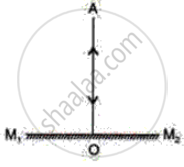What are the angles of incidence and reflection?

Reflection of Light | Q 36 | Page 247
State two main characteristics of the image formed by a plane mirror.
Exercise | Q 37 | Page 247

Draw two sets of rays of light to show the formation of an image of the letter as shown in figure p.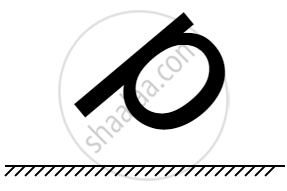Exercise | Q 39 | Page 247

Show an incident ray AO and the normal ON a plan mirror. draw the reflected ray and then find the angle between the incident and reflected rays.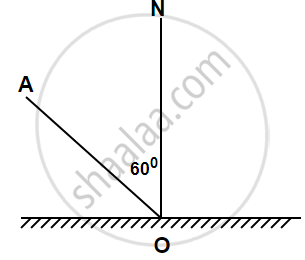Spherical Mirrors, Exercise [Page 258]

### Frank solutions for Class 9 Physics ICSE Chapter 6 Light Spherical Mirrors, Exercise [Page 258]

Spherical Mirrors | Q 1 | Page 258
What do you mean by a spherical mirror?
Spherical Mirrors | Q 2 | Page 258
Explain with a suitable diagram, the converging of a parallel beam of light rays by a concave mirror.
Spherical Mirrors | Q 3 | Page 258
If the radius of curvature of a concave mirror is 30 cm, what is its focal length?
Spherical Mirrors | Q 4 | Page 258
What do you mean by a focal point?
Spherical Mirrors | Q 5 | Page 258
Distinguish between real and virtual images.
Spherical Mirrors | Q 6.1 | Page 258

Define the term Pole.

Spherical Mirrors | Q 6.2 | Page 258

Define the term Centre of curvature.

Spherical Mirrors | Q 6.3 | Page 258

Define the term Aperture.

Spherical Mirrors | Q 6.4 | Page 258

Define the term Principal axis.

Spherical Mirrors | Q 6.5 | Page 258

Define the term Principal focus.

Spherical Mirrors | Q 7 | Page 258
Which mirror has a wider field of view?
Spherical Mirrors | Q 8 | Page 258
Give three applications of concave mirrors.
Spherical Mirrors | Q 9 | Page 258
Mention one main application of convex mirrors.
Spherical Mirrors | Q 10 | Page 258
What kind of mirror is used in vehicles to see the traffic following it?
Spherical Mirrors | Q 11 | Page 258

What is the relationship between the focal length and radius of curvature?

Spherical Mirrors | Q 12 | Page 258
For what position of an object, a concave mirror forms a real image equal in size to the object?
Spherical Mirrors | Q 13 | Page 258
For what position of an object, a concave mirror forms an enlarged virtual image?
Spherical Mirrors | Q 14 | Page 258
Name the spherical mirror which can produce a real and diminished image of the object.
Spherical Mirrors | Q 15 | Page 258
What is the focal length of a plane mirror?
Spherical Mirrors | Q 16 | Page 258
Where should an object be placed in front of a concave mirror so as obtain its magnified erect image?
Spherical Mirrors | Q 17 | Page 258
How will you determine the focal length of a concave mirror?
Spherical Mirrors | Q 18 | Page 258
What do you mean by the magnification produced by mirrors?
Spherical Mirrors | Q 19 | Page 258
Write down the SI unit of focal length.
Spherical Mirrors | Q 20 | Page 258
A person in front of a spherical mirror finds his image having a very small head, a fat body and legs of normal size. What are the shapes of three parts of the mirror?
Spherical Mirrors | Q 21 | Page 258
What is the nature of a mirror having a focal length of +15 cm?
Spherical Mirrors | Q 22 | Page 258
What is the nature of the mirror having a focal length of -20 cm?
Spherical Mirrors | Q 23 | Page 258
When we look into a plane mirror, is the image of our face real or virtual?
Spherical Mirrors | Q 24 | Page 258
An object is brought towards a concave mirror. How does the position and size of the image change?
Exercise | Q 25 | Page 258

AB is the object, A1B1 is its image. MM' is the position of the mirror. Complete the ray diagram and find the position of the center of curvature and focus of the mirror. Also, measure the focal length.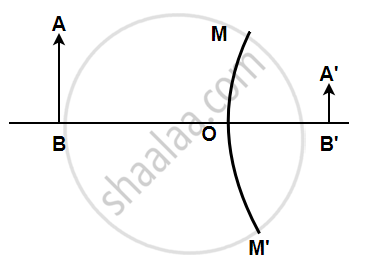Exercise | Q 26 | Page 258

AB is the object, A'B' is the image, and MM' is the position of the mirror. Complete the ray diagram showing the formation of the image and find the focal length of the mirror.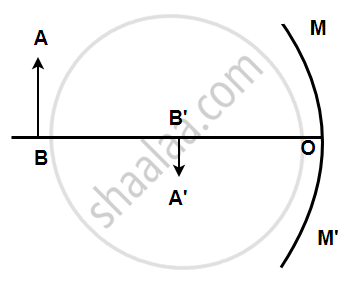Exercise | Q 27.1 | Page 258

Complete the following diagrams shown in the following figures by drawing the reflected rays.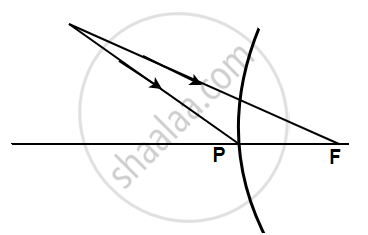Exercise | Q 27.2 | Page 258

Complete the following diagrams shown in the following figures by drawing the reflected rays.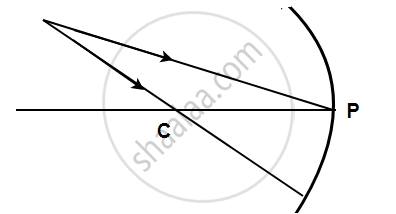Exercise | Q 28.1 | Page 258

Complete the following diagrams shown in the below figure by drawing the reflected ray for each incident ray.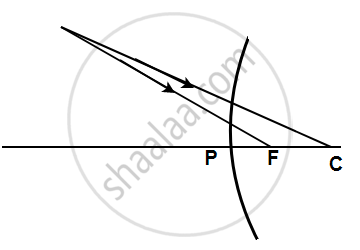Exercise | Q 28.2 | Page 258

Complete the following diagrams shown in the below figure by drawing the reflected ray for each incident ray.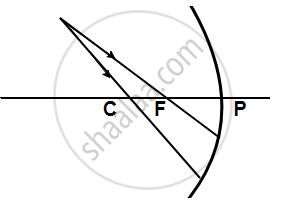Exercise | Q 29 | Page 258

The following figure shows a concave mirror with its pole (P), focus (F), and center of curvature (C). Draw a ray diagram to show the formation of the image of an object AB by the concave mirror.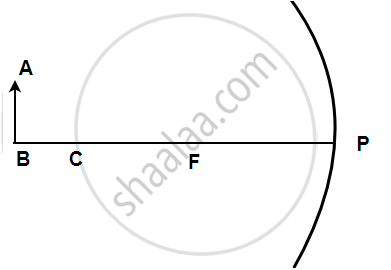Exercise | Q 30 | Page 258

In the following figure shows a concave mirror with its pole (P), focus (F) and centre of curvature (C). Draw a ray diagram to show the formation of the image of an object AB by the concave mirror.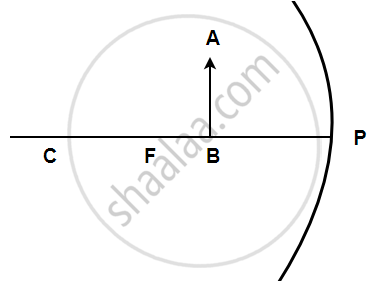Exercise [Pages 261 - 264]

### Frank solutions for Class 9 Physics ICSE Chapter 6 Light Exercise [Pages 261 - 264]

Exercise | Q 1 | Page 261
Name the mirror having a wider field of view.
Exercise | Q 2 | Page 261
Name the mirror which always produces an erect image of the objects.
Exercise | Q 3 | Page 261
What kind of mirror is used in vehicles to see the traffic on the rear side?
Exercise | Q 4 | Page 261
If you want to see an enlarged image of your face, state whether you will use a convex mirror or a concave mirror?
Exercise | Q 5 | Page 261
An object is placed at a long distance in front of a convex mirror of radius of curvature 20 cm. State the position of the image.
Exercise | Q 5 | Page 261
Name the spherical mirror which can produce a real and diminished image of an object.
Exercise | Q 6 | Page 261

Name the spherical mirror which can produce a real and diminished image of an object.

Exercise | Q 7 | Page 261
What do you mean by the focal length of a mirror?
Exercise | Q 8 | Page 261
Name the type of mirror having a focal length of +20 cm.
Exercise | Q 9 | Page 261
What is the focal length of a plane mirror?
Exercise | Q 10 | Page 261
Name the type of mirror having a focal length of -15 cm.
Exercise | Q 11 | Page 261
What do you mean by the principal axis?
Exercise | Q 12 | Page 261
Define linear magnification of a mirror.
Exercise | Q 13 | Page 261
Define pole of a mirror.
Exercise | Q 14 | Page 261
What do you mean by centre of curvature of a mirror?
Exercise | Q 15 | Page 261
State three characteristics of light.
Exercise | Q 16 | Page 261
Give three distinctions between sound and light waves.
Exercise | Q 17 | Page 261
State three characteristics of the image formed by a concave mirror.
Exercise | Q 18 | Page 261
State three characteristics of the image formed by a convex mirror.
Exercise | Q 19 | Page 261
Give three uses of concave mirrors.
Exercise | Q 20 | Page 261
Write the sign convection used in optics.
Exercise | Q 21.1 | Page 261

What is a mirror formula?

Exercise | Q 21.2 | Page 261

Define linear magnification produced by a mirror?

Exercise | Q 22 | Page 261

Find the height of the image of a body of height 1.5m in a mirror with a magnification of 1.5.

Exercise | Q 23 | Page 261
Define linear magnification. Does it have any units? Write down a formula for the magnification produced by a concave mirror in terms of image distance and object distance.
Exercise | Q 24 | Page 261
Distinguish between real and virtual images.
Exercise | Q 25 | Page 261
Distinguish between regular and irregular reflection.
Exercise | Q 26.1 | Page 261
What do you mean by reflection?
Exercise | Q 26.2 | Page 261
State the laws of reflection.
Exercise | Q 27 | Page 261
How will you distinguish between a plane mirror, a concave mirror and a convex mirror without touching them?
Exercise | Q 28 | Page 261
How can you distinguish between a convex mirror and a concave mirror? Explain.
Exercise | Q 29 | Page 262

The boxes in figure (a, b, c) represent mirrors; insert a mirror which will reflect the incident ray as shown in the diagram.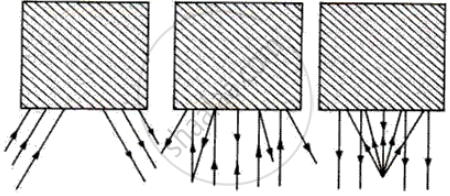Exercise | Q 30 | Page 262

Give two uses of convex mirror.

Exercise | Q 31 | Page 262
You can see the reflection of your clearly on a polished table-top is not polished. Explain.
Exercise | Q 32.1 | Page 262

State whether the following statement is true or false. If false, correct it.

The angle of incidence is the angle made by the incident ray with the plane mirror.

• True

• False

Exercise | Q 32.2 | Page 262

State whether the following statement is true or false. If false, correct it.

If a ray of light incident on a plane mirror is such that it makes an angle of 30o with the mirror, then the angle of reflection is 60o.

• True

• False

Exercise | Q 32.3 | Page 262

State whether the following statement is true or false. If false, correct it.

If the incident ray makes an angle of Xo with the normal, then the reflected ray is 2Xo.

• True

• False

Exercise | Q 32.4 | Page 262

State whether the following statement is true or false. If false, correct it.

The image in a plane mirror is situated in the mirror.

• True

• False

Exercise | Q 32.5 | Page 262

State whether the following statement is true or false. If false, correct it.

The image formed in a plane mirror is the real, erect, and same size as that of the object.

• True

• False

Exercise | Q 33 | Page 262

When a ray of light strikes a plane mirror at an angle of 150 with the mirror, what will be the angle through which the ray will deviate? Illustrate with a ray diagram in the following figure.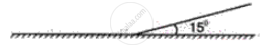Exercise | Q 34 | Page 262
You are provided with two plane mirrors. Draw a ray diagram to show how the ray of light can be turned through an angle of 180°.
Exercise | Q 35 | Page 262
What are the characteristics of the image formed by a plane mirror?
Exercise | Q 36.1 | Page 262

The word PLATE written on a paper is held in front of a plane mirror. Write down the letters as seen in the following case.
The paper is held parallel to the mirror.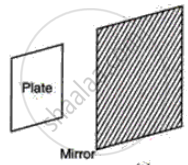Exercise | Q 36.2 | Page 262

The word PLATE written on a paper is held in front of a plane mirror. Write down the letters as seen in the following case.
The paper is held perpendicular to the mirror.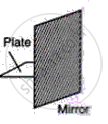Exercise | Q 37 | Page 262

A boy is standing in front of a plane mirror at a distance of 3 m from it.
(i) What is the distance between the boy and his image?
(ii) If the boy moves 1 m backward, find the distance between the image and the boy.

Exercise | Q 38 | Page 262
Draw a neat and labeled ray diagram of a simple periscope. Give two uses of a periscope.
Exercise | Q 39.1 | Page 263

Complete the ray diagram to show the image observed by the eye after reflection in the following figure.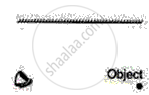Exercise | Q 39.2 | Page 263

Complete the ray diagram to show the image observed by the eye after reflection in the following figure.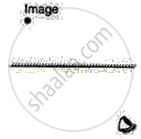Exercise | Q 40.1 | Page 263

Define the term Pole.

Exercise | Q 40.2 | Page 263

Define the term Centre of curvature.

Exercise | Q 40.3 | Page 263

Define the term Principle focus.

Exercise | Q 40.4 | Page 263

Define the term Principle axis.

Exercise | Q 40.5 | Page 263

Define the term Focus of a concave mirror.

Exercise | Q 40.6 | Page 263

Define the term Normal.

Exercise | Q 41.1 | Page 263

Locate the image termed when the object is placed between two planes as shown in the following figure.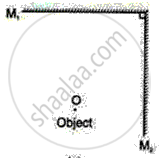Exercise | Q 41.2 | Page 263

Locate the image termed when the object is placed between two planes as shown in the following figure.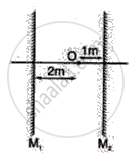Exercise | Q 42.1 | Page 263

Complete the path of incident ray in the following case.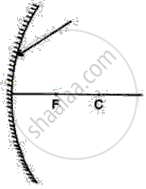Exercise | Q 42.2 | Page 263

Complete the path of incident ray in the following case.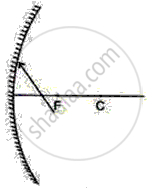Exercise | Q 42.3 | Page 263

Complete the path of incident ray in the following case.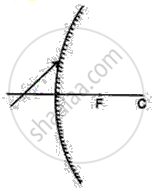Exercise | Q 43 | Page 263

By geometrical construction, find the position of the image in the following figure.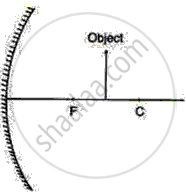Exercise | Q 44 | Page 263

An object is placed in front of a convex mirror of focal length 25 cm. By scale, a drawing shows the formation of image and states the nature of image.
Given that v = 10 cm.

Exercise | Q 45.1 | Page 263

Draw a ray diagram to show that a convex mirror has a wider field of view.

Exercise | Q 45.2 | Page 263

A concave mirror can be used to produce a parallel beam of light. Draw a ray to illustrate this.

Exercise | Q 46.1 | Page 263

An object 10 cm high is placed at a distance of 20 cm in front of a spherical mirror of focal length 25 cm. By scale drawing find the nature, position, and magnification of the image in the following case:
Concave mirror

Exercise | Q 46.2 | Page 263

An object 10 cm high is placed at a distance of 20 cm in front of a spherical mirror of focal length 25 cm. By scale drawing find the nature, position, and magnification of the image in the following case:
Convex mirror

Exercise | Q 47 | Page 264

An object is placed in front of a concave mirror as shown in the following figure. By scale drawing, find the nature of the image. Given f = 10 cm, v = 30 cm.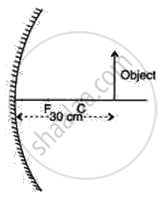Exercise | Q 48 | Page 264
An object 5 cm in height is placed at a distance of 30 m in front of a concave mirror of focal length 40 cm. By scale, drawing finds the nature, size, position, and magnification of the image.
Exercise | Q 49 | Page 264

The following Figure shows a concave mirror MM' on which a ray of light incident from a point P gets reflected to meet the principle axis at O.
(a) Find, by construction, the position of the centre of curvature of the concave mirror.
(b) Write down the value for the radius of curvature of the mirror.
(c) Calculate the focal length of the mirror.
(d ) Which relation is used in deducing the focal length from the radius of curvature?

Exercise | Q 50 | Page 264

The diagram below shows the parallel rays incident on a convex mirror. C is the centre of the curvature of the mirror. By drawing the paths of the reflected rays, label the focus F and hence find the focal length of the mirror.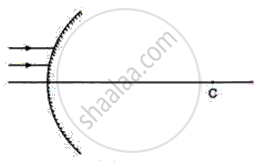## Chapter 6: Light

Reflection of LightExerciseSpherical Mirrors## Frank solutions for Class 9 Physics ICSE chapter 6 - Light

Frank solutions for Class 9 Physics ICSE chapter 6 (Light) include all questions with solution and detail explanation. This will clear students doubts about any question and improve application skills while preparing for board exams. The detailed, step-by-step solutions will help you understand the concepts better and clear your confusions, if any. Shaalaa.com has the CISCE Class 9 Physics ICSE solutions in a manner that help students grasp basic concepts better and faster.

Further, we at Shaalaa.com provide such solutions so that students can prepare for written exams. Frank textbook solutions can be a core help for self-study and acts as a perfect self-help guidance for students.

Concepts covered in Class 9 Physics ICSE chapter 6 Light are Rules for the Construction of Image Formed by a Spherical Mirror, Focus and Focal Length, Images Formed by Spherical Mirrors, Concave Mirror, Image Formation by Concave Mirror, Convex Mirror, Mirror equation/Formula, Images Formed by a Plane Mirrors, Distinction Between a Plane Mirror, Concave Mirror and Convex Mirror, Images Formed in a Pair of Mirrors Placed Parallel to Each Other, Images Formed by Two Mirrors Placed Perpendicular to Each Other, Spherical Mirrors, Image Formation by Convex Mirror, Relationship Between the Focal Length and Radius of Curvature, Sign Convention for Reflection by Spherical Mirrors, Reflection of Light, Types of Reflection, Terms Used in Reflection of Light, Law of Reflection of Light, Verification of the Law of Reflection of Light, Formation of Image by Reflection: Real and Virtual Image, Formation of Image of a Point Object by a Plane Mirror, Image of an Extended Object Formed by a Plane Mirror, Position of Image, Lateral Inversion, Plane Mirror and Reflection, Images Formed in Two Inclined Mirrors.

Using Frank Class 9 solutions Light exercise by students are an easy way to prepare for the exams, as they involve solutions arranged chapter-wise also page wise. The questions involved in Frank Solutions are important questions that can be asked in the final exam. Maximum students of CISCE Class 9 prefer Frank Textbook Solutions to score more in exam.

Get the free view of chapter 6 Light Class 9 extra questions for Class 9 Physics ICSE and can use Shaalaa.com to keep it handy for your exam preparation